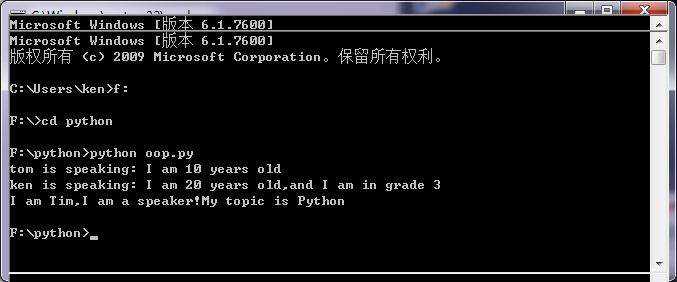# Python类定义和类继承

## 类的定义

``````class <类名>:
<语句>``````

## 类的私有属性

__private_attrs  两个下划线开头，声明该属性为私有，不能在类地外部被使用或直接访问，在类内部的方法中使用时 self.__private_attrs。

## 类的方法

### 类的私有方法

__private_method 两个下划线开头，声明该方法为私有方法，不能在类地外部调用,在类的内部调用slef.__private_methods

### 类的专有方法

• __init__ ： 构造函数，在生成对象时调用
• __del__  ： 析构函数，释放对象时使用
• __repr__： 打印，转换
• __setitem__： 按照索引赋值
• __getitem__： 按照索引获取值
• __len__： 获得长度
• __cmp__： 比较运算
• __call__： 函数调用
• __sub__： 减运算
• __mul__： 乘运算
• __div__： 除运算
• __mod__： 求余运算
• __pow__： 乘方

``````#类定义
class people:
#定义基本属性
name = ''
age = 0
#定义私有属性,私有属性在类外部无法直接进行访问
__weight = 0
#定义构造方法
def __init__(self,n,a,w):
self.name = n
self.age = a
self.__weight = w
def speak(self):
print("%s is speaking: I am %d years old" %(self.name,self.age))

p = people('tom',10,30)
p.speak()``````

## 类的继承

### 单继承

``````class <类名>(父类名)
<语句>
eg.
class childbook(book)
age = 10``````

### 多重继承

``````class 类名(父类1,父类2,....,父类n)
<语句1>``````

``````#另一个类，多重继承之前的准备
class speaker():
topic = ''
name = ''

def __init__(self,n,t):
self.name = n
self.topic = t

def speak(self):
print("I am %s,I am a speaker!My topic is %s"%(self.name,self.topic))

#多重继承
class sample(speaker,student):
a =''

def __init__(self,n,a,w,g,t):
student.__init__(self,n,a,w,g)
speaker.__init__(self,n,t)

test = sample("Tim",25,80,4,"Python")
test.speak()#方法名同，默认调用的是在括号中排前地父类的方法``````

### 类方法的覆写

``````import A
l = A.func1()``````